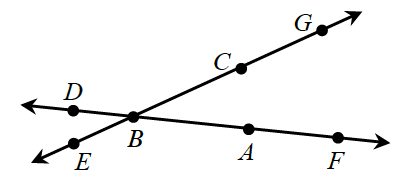### Home > CCG > Chapter 1 > Lesson 1.2.3 > Problem1-78

1-78.

Examine the diagram at right. Which angle below is another name for $∠ABC$? Note: More than one solution is possible.1. $∠ABE$

1. $∠GBD$

1. $∠FBG$

1. $∠EBC$

1. none of these

Trace the points in order to see if they make the same angle as $\angle ABC$.

C